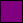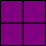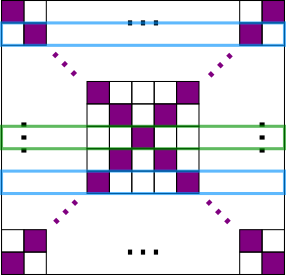You may also likeDays and Dates

Investigate how you can work out what day of the week your birthday will be on next year, and the year after...Summing Consecutive Numbers

15 = 7 + 8 and 10 = 1 + 2 + 3 + 4. Can you say which numbers can be expressed as the sum of two or more consecutive integers?1 Step 2 Step

Liam's house has a staircase with 12 steps. He can go down the steps one at a time or two at time. In how many different ways can Liam go down the 12 steps?

Tiled Floor

Age 11 to 14 ShortChallenge Level

The sequence of all floorsEven floors: 2 purple tiles per row (total number even)
Odd floors: 1 more purple tile than the previous floor (total number odd)109 purple tiles
108 = 2$\times$54 so floor length 54 has 108 purple tiles
$\therefore$ floor length 55 has 108 + 1 = 109 purple tiles
Floor length 55 has 55$^2$ = 3025 tiles

The sequence of odd-sided floors
Each odd-sided floor contains the last odd-sided floor in the middle, outlined in blue.4 purple tiles are added each time
Side length 2 longer each time

109 = 1 + 4$\times$?
= 1 + 108
= 1 + 4$\times$27

So side length = 1 + 2$\times$27 = 55

$\therefore$ total number of tiles = 55$^2$ = 3025

Finding the number of rowsThere are 2 purple tiles on each row,
Except for the middle row which has 1 purple tile (on odd-sided floors)

109 tiles = 1 purple tile in the middle
+ 108 purple tiles in pairs
= 1 purple tile in the middle
+ 54 pairs on 54 rows

$\therefore$ there are 1 + 54 = 55 rows
So there are 55$^2$ = 3025 tiles

You can find more short problems, arranged by curriculum topic, in our short problems collection.